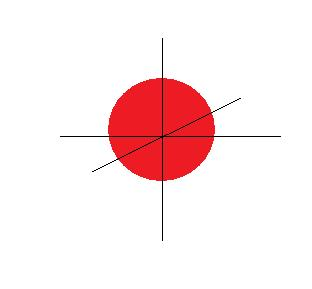# 1.6A: Electronic Quantum Numbers

$$\newcommand{\vecs}{\overset { \rightharpoonup} {\mathbf{#1}} }$$ $$\newcommand{\vecd}{\overset{-\!-\!\rightharpoonup}{\vphantom{a}\smash {#1}}}$$$$\newcommand{\id}{\mathrm{id}}$$ $$\newcommand{\Span}{\mathrm{span}}$$ $$\newcommand{\kernel}{\mathrm{null}\,}$$ $$\newcommand{\range}{\mathrm{range}\,}$$ $$\newcommand{\RealPart}{\mathrm{Re}}$$ $$\newcommand{\ImaginaryPart}{\mathrm{Im}}$$ $$\newcommand{\Argument}{\mathrm{Arg}}$$ $$\newcommand{\norm}{\| #1 \|}$$ $$\newcommand{\inner}{\langle #1, #2 \rangle}$$ $$\newcommand{\Span}{\mathrm{span}}$$ $$\newcommand{\id}{\mathrm{id}}$$ $$\newcommand{\Span}{\mathrm{span}}$$ $$\newcommand{\kernel}{\mathrm{null}\,}$$ $$\newcommand{\range}{\mathrm{range}\,}$$ $$\newcommand{\RealPart}{\mathrm{Re}}$$ $$\newcommand{\ImaginaryPart}{\mathrm{Im}}$$ $$\newcommand{\Argument}{\mathrm{Arg}}$$ $$\newcommand{\norm}{\| #1 \|}$$ $$\newcommand{\inner}{\langle #1, #2 \rangle}$$ $$\newcommand{\Span}{\mathrm{span}}$$$$\newcommand{\AA}{\unicode[.8,0]{x212B}}$$

## Introduction

There are four quantum numbers ($$n$$, $$l$$, $$m_{l}$$, $$m_{s}$$). No two electrons in the same atom can have the same four quantum numbers. Each quantum number describes a different aspect of the electron and its orbital. These numbers are obtained from the solution of the Schrödinger Equation for atoms in spherical coordinates.

## n, the principal quantum number

$$n$$tells you about the size of the orbital. It is related to how far the electron is from the atom. It is also related to the energy of the electron. $$n$$can be any positive integer number. An orbital has $$n-1$$radial nodes as well, which describe a radius at which the probability of finding the electron is 0.

## l, azimuthal quantum number

lt tells you about the angular momentum of the electron in the orbital. It defines the shape of the orbital. lt can be any integer between (and including) 0 and ($$n-1$$). Each orbital has $$l$$planar nodes.
Some examples of orbitals with different $$l$$:

$$l=0$$is an s orbital, with no planar nodes.$$l=1$$gives a p orbital, which has one planar node.## ml, magnetic quantum number

$$m_{l}$$ tells you about the angular momentum projected on to the z axis. It tells you of the orientation of the orbital. It can be any integer between $$-l$$ and $$l$$.

$$l=1$$ has 3 different (but degenerate) $$m_{l}$$ possible values:-1,0 or 1.

$$p_{x}$$$$p_{y}$$$$p_{z}$$## ms spin projection quantum number

$$m_{s}$$tells you about the spin of the electron. An electron is a fermion, a type of quantum particle which is only allowed to have $$m_{s}$$equal to -1/2 or 1/2.

## Pauli Exclusion Principle

The Pauli Exclusion principle states that no two identical fermions can share the same quantum state simultaneously. An electron, being a fermion, obeys this principle, so no two electrons in the same atom can have the same quantum numbers. Each orbital has space for 2 electrons, with $$m_{s}$$ values with opposite sign. These electrons are considered spin-paired.

## Energies of the Orbitals

The energies of the orbitals depend solely on $$n$$ and $$l$$ so there are degenerate states that result from various $$m_{l}$$ values. For example, there are 3 p orbitals, all of which are equal in energy and therefore degenerate. Here is a table of possible quantum number arrangements and the corresponding orbitals from $$n=1$$ to $$n=5$$:

$$n$$ $$l$$ $$m_{l}$$ $$m_{s}$$ Orbital
$$1$$ $$0$$ $$0$$ $$\pm \frac{1}{2}$$ $$1s$$
$$2$$ $$0$$ $$0$$ $$\pm \frac{1}{2}$$ $$2s$$
$$2$$ $$1$$ $$\pm 1$$ or $$0$$ $$\pm \frac{1}{2}$$ $$2p_{x}, 2p_{y} \; or \; 2p_{z}$$
$$3$$ $$0$$ $$0$$ $$\pm \frac{1}{2}$$ $$3s$$
$$3$$ $$1$$ $$\pm 1$$ or $$0$$ $$\pm \frac{1}{2}$$ $$3p_{x}, 3p_{y} \; or \; 3p_{z}$$
$$3$$ $$2$$ $$\pm 2, \pm 1$$ or $$0$$ $$\pm \frac{1}{2}$$ $$3d_{z^2}, 3d_{x^2-y^2}, 3d_{xy},$$$$3d_{xz}\; or \; 3d_{yz}$$
$$4$$ $$0$$ $$0$$ $$\pm \frac{1}{2}$$ $$4s$$
$$4$$ $$1$$ $$\pm 1$$ or $$0$$ $$\pm \frac{1}{2}$$ $$4p_{x}, 4p_{y} \; or \; 4p_{z}$$
$$4$$ $$2$$ $$\pm 2, \pm 1$$ or $$0$$ $$\pm \frac{1}{2}$$ $$4d_{z^2}, 4d_{x^2-y^2}, 4d_{xy},$$$$4d_{xz} \; or \; 4d_{yz}$$
$$4$$ $$3$$ $$\pm 3, \pm 2, \pm 1$$ or $$0$$ $$\pm \frac{1}{2}$$ $$4f_{x^3}, 4f_{y^3}, 4f_{z^3},$$$$4f_{x (z^2-y^2)}, 4f_{y (z^2-x^2)},$$$$4f_{z (x^2-y^2)}or \; 4f_{xyz}$$
$$5$$ $$0$$ $$0$$ $$\pm \frac{1}{2}$$ $$5s$$
$$5$$ $$1$$ $$\pm 1$$or $$0$$ $$\pm \frac{1}{2}$$ $$5p_{x}, 5p_{y} \; or \; 5p_{z}$$
$$5$$ $$2$$ $$\pm 2, \pm 1$$ or $$0$$ $$\pm \frac{1}{2}$$ $$5d_{z^2}, 5d_{x^2-y^2}, 5d_{xy}, 5d_{xz} \; or \; 5d_{yz}$$
$$5$$ $$3$$ $$\pm 3, \pm 2, \pm 1$$ or $$0$$ $$\pm \frac{1}{2}$$ $$5f_{x^3}, 5f_{y^3}, 5f_{z^3}, 5f_{x (z^2-y^2)}, 5f_{y (z^2-x^2)}, 5f_{z (x^2-y^2)} or \; 5f_{xyz}$$
$$5$$ $$4$$ $$\pm 4, \pm 3, \pm 2, \pm 1$$ or $$0$$ $$\pm \frac{1}{2}$$ $$5g_{z^4}, 5g_{z^3 x}, 5g_{z^3 y}, 5g_{z^{2} xy}, 5g_{z^2 (x^2-y^2)}, 5g_{zx^3}, 5g_{zy^3}, 5g_{xy (x^2-y^2)} \; or 5g_{x^4+y^4}$$

## References

1. J Goldstone, F Wilczek, Fractional quantum numbers on solitons - Physical Review Letters, 1981 - APS
2. Harris, R. ,2007, Modern Physics, 2e, Addison Wesley